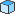# Altova FlowForce Server 2020 Advanced Edition

 FlowForce Expressions Operators Scroll Home Prev Top Next More

To build FlowForce expressions, you can use the operators listed below. Remember that you can test any expression by calling the built-in functionsystem/compute.

Operator

Description

Example

==

Checks if a and b are equal (numerically equal for numbers, code-point equal for strings).

== 5 computes to true

2 + 3 == 4 computes to false

!=

Checks if a and b are not equal. Note that the following three expressions are equivalent:

a != b

not (a == b)

a <> b

+ 2 != 5 computes to true

+ 2 != 5 computes to false

<

Checks if a is less than b (numerically less for numbers, see below for strings).

computes to true

<=

Checks if a is less than or equal to b.

5 <=  computes to true

>

Checks if a is greater than b.

5 > 1  computes to true

>=

Checks if a is greater than or equal to b.

5 >=  computes to true

+

1 computes to 2

-

Subtraction.

1 computes to 1

*

Multiplication.

3 *  computes to 6

/

Division.

6 / 3 computes to 2

String comparisons are performed as follows:

The common prefix of the two strings are ignored (evaluated on code points)

If both remaining strings are non-empty, their first code points are compared numerically

Empty strings are less than non-empty strings

Use parentheses to instruct FlowForce to evaluate the expression inside first. For example:

2 + 3 * 4 computes to 14.

(2 + 3) * 4 computes to 20.